Courses

# Maxwell's Equations and Displacement Current Class 12 Notes | EduRev

## Class 12 : Maxwell's Equations and Displacement Current Class 12 Notes | EduRev

The document Maxwell's Equations and Displacement Current Class 12 Notes | EduRev is a part of the Class 12 Course Physics Class 12.
All you need of Class 12 at this link: Class 12

Electromagnetic Waves

Displacement Current

It is a current which produces in the region in which the electric field and hence the electric flux changes with time.

Displacement current, ID = εo . dφE / dt

where, φE is the electric flux.

Ampere-Maxwell Law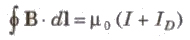where, μo = Permeability

= 4π * 10-7 V / Am

Maxwell’s Equations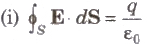This equation is Gauss’s law in electrostatics.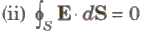This equation is Gauss's law in magnetostatics.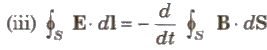This equation is Faraday’s law of electromagnetic induction.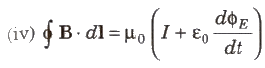This equation is Ampere-Maxwell law.

Offer running on EduRev: Apply code STAYHOME200 to get INR 200 off on our premium plan EduRev Infinity!

,

,

,

,

,

,

,

,

,

,

,

,

,

,

,

,

,

,

,

,

,

;